Courses

# Baics of Inverse Trigonometric Functions Mathematics Notes | EduRev

## Mathematics : Baics of Inverse Trigonometric Functions Mathematics Notes | EduRev

The document Baics of Inverse Trigonometric Functions Mathematics Notes | EduRev is a part of the Mathematics Course Additional Topics for IIT JAM Mathematics.
All you need of Mathematics at this link: Mathematics

The inverse trigonometric functions are the inverse functions of the trigonometric functions, written cos-1z, cot-1z, csc-1z, sec-1z, sin-1z, and tan-1z.
Alternate notations are sometimes used, as summarized in the following table.

 f(z) alternate notations cos-1 z arccos z (Spanier and Oldham 1987, p. 333; Gradshteyn and Ryzhik 2000, p. 207) cot-1 z arccot z (Spanier and Oldham 1987, p. 333), arcctg z (Spanier and Oldham 1987, p. 333; Gradshteyn and Ryzhik 2000, p. 208; Jeffrey 2000, p. 127) csc-1 z arccsc z (Spanier and Oldham 1987, p. 333), arccosec z (Spanier and Oldham 1987, p. 333; Gradshteyn and Ryzhik 2000, p. 207) sec-1 z arcsec z (Spanier and Oldham 1987, p. 333; Gradshteyn and Ryzhik 2000, p. 209) sin-1 z arcsin z (Spanier and Oldham 1987, p. 333; Gradshteyn and Ryzhik 2000, p. 207) tan-1 z arctan z (Spanier and Oldham 1987, p. 333), arctg z (Spanier and Oldham 1987, p. 333; Gradshteyn and Ryzhik 2000, p. 208; Jeffrey 2000, p. 127)

The inverse trigonometric functions are multivalued. For example, there are multiple values of w such that z=sinw, so sin-1 z is not uniquely defined unless a principal value is defined. Such principal values are sometimes denoted with a capital letter so, for example, the principal value of the inverse sine sin-1 z may be variously denoted Sin-1 z or Arcsin z (Beyer 1987, p. 141). On the other hand, the notation sin-1 z (etc.) is also commonly used denote either the principal value or any quantity whose sine is z an (Zwillinger 1995, p. 466).
Worse still, the principal value and multiply valued notations are sometimes reversed, with for example arcsinz denoting the principal value and Arcsinz denoting the multivalued functions (Spanier and Oldham 1987, p. 333).
Since the inverse trigonometric functions are multivalued, they require branch cuts in the complex plane. Differing branch cut conventions are possible, but those adopted in this work follow those used by the Wolfram Language, summarized below.

 function name function Wolfram Language branch cut(s) inverse cosecant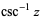ArcCsc[z] (-1, 1) inverse cosine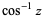ArcCos[z]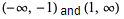inverse cotangent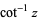ArcCot[z] (-i, i) inverse secant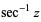ArcSec[z] (-1, 1) inverse sine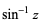ArcSin[z]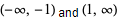inverse tangent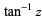ArcTan[z]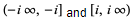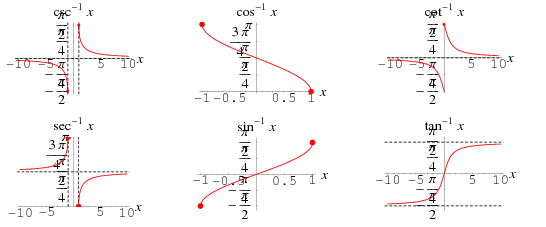Different conventions are possible for the range of these functions for real arguments. Following the convention used by the Wolfram Language, the inverse trigonometric functions defined in this work have the following ranges for domains on the real line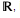illustrated above.

 function name function domain range inverse cosecant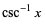(-∞, ∞)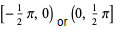inverse cosine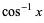[-1, 1]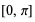inverse cotangent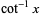(-∞, ∞)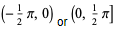inverse secant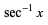(-∞, ∞)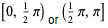inverse sine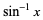[-1, 1]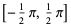inverse tangent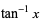(-∞, ∞)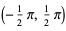Inverse-forward identities are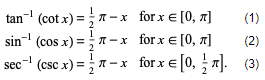Forward-inverse identities are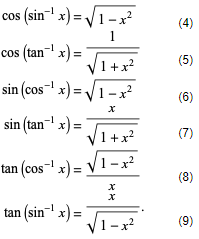Inverse sum identities include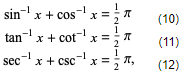where equation (11) is valid only for x >0.
Complex inverse identities in terms of natural logarithms include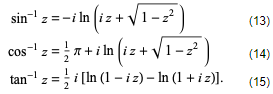Offer running on EduRev: Apply code STAYHOME200 to get INR 200 off on our premium plan EduRev Infinity!

40 docs

,

,

,

,

,

,

,

,

,

,

,

,

,

,

,

,

,

,

,

,

,

;